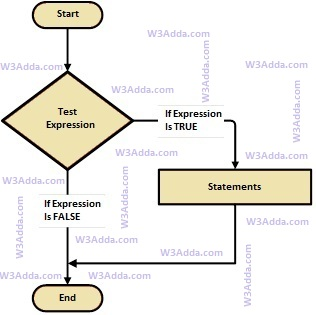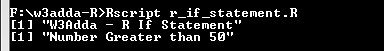# R If Statement

In this tutorial you will learn about the R If Statement and its application with practical example.

## R if Statement

In R, If statement allows a block of code to be executed only when a specified condition is true. An if statement evaluates a boolean expression followed by one or more statements. The given boolean expression results in a boolean value that can only be either true or false.

## R If Statement Flow DiagramR-if-statement

Syntax:-

Here, condition is a Boolean expression that results in either True or False, if it results in True then statements inside if body are executed, if it results in False then execution is skipped from if body.

Example:-

Output:-In this tutorial we have learn about the R If Statement and its application with practical example. I hope you will like this tutorial.